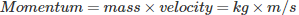# MCQs on Electrical And Electronics Measurements

##### Page 1 of 19. Go to page 1 2 3 4 5 6 7 8 9 10 11 12 13 14 15 16 17 18 19
01․ Which of the following quantities consists of S.I. unit as Hertz?
Charge
Force
Frequency
Power

Frequency defined as the number of cycles per second and discovered by German physicist Heinrich Hertz. The S.I. unit of frequency is Hertz.

02․ Which one of the following units is a fundamental unit?
Newton
Ampere
Watt
Joule/sec

The ampere is the basic unit specifically in the SI unit system of measurement but other systems of measurement use different base units. Newton, Watt and Joules/sec are constructed by more than one fundamental unit.

03․ Which of the quantity consists of SI unit as Candela?
Velocity
Impulse
Luminous intensity
Force

Candela is the unit of luminous intensity. Luminous intensity is the amount of light emitting from a point source per unit of solid angle from a point source.

04․ Which of the quantity consists of unit as Pascal ?
Temperature
Pressure
Force
Impulse

Pascal is the SI unit for pressure named after Blaise Pascal, French mathematician. Force per unit area is known as pressure.

05․ Which of the quantity consists of unit as newton-second?
Impulse
Acceleration
Speed
velocity

The SI unit of impulse is called Newton second (N-s). Integral of force over a time is called impulse.

06․ Which of the quantity consists of unit as kg m/sec ?
Speed
Momentum
Acceleration
Impulse

The kilogram-meter per second is the standard unit of momentum. It is the product of mass and velocity.07․ What is the standard unit for length ?
Meter
Inch
Kilometer
Centimeter

The basis of length units for the metric system is the meter. It is the international standard SI unit of length.

08․ What is the name of physical quantities which are independent of each other?
Fundamental quantity
Derived quantity
Numerical quantity
None of the above

The fundamental quantities are those which are independent and are not derived from other quantities example length, mass, temperature etc.

09․ The Metric System is also called as:
CGS
MKS system
SI
None of the above

The metric system uses the three base units: Length, Mass, Volume. MKS system (Meter, Kilogram, Second).

10․ Which devices used to measure the temperature of an object ?
Poteintiometer
Odometer
Thermometers
Galvanometer

A thermometer is a device that measures temperature or temperature gradient.

123>>>